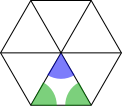#### You may also like### Building Tetrahedra

Can you make a tetrahedron whose faces all have the same perimeter?A 1 metre cube has one face on the ground and one face against a wall. A 4 metre ladder leans against the wall and just touches the cube. How high is the top of the ladder above the ground?Four rods are hinged at their ends to form a convex quadrilateral. Investigate the different shapes that the quadrilateral can take. Be patient this problem may be slow to load.

# Ratio of Areas

##### Age 14 to 16 ShortChallenge LevelThree methods are shown below, but all of them use this idea.

If you draw lines from the centre of the hexagon to each of its vertices, 6 identical isosceles triangles are formed (with the green angles equal).

The blue angle is 360$^\circ\div$6 = 60$^\circ$, which means that the green angles must also be 60$^\circ$, and so the hexagon is split into 6 equilateral triangles - which have side length 1, the same as the hexagon.

Splitting both shapes into equilateral triangles with side length 1We have seen that we can split the hexagon into 6 equilateral triangles with side length 1. We can try to do the same to the triangle.

Joining the midpoints of the sides, as shown on the right, we can split the triangle into 4 equilateral triangles with side length 2.Doing the same to each of the smaller triangles formed gives equilateral triangles with side length 1. There are 4$\times$4 = 16 of these in the large equilateral triangle.

So the hexagon contains 6 equilateral triangles of side length 1, whilst the triangle contains 16. So their areas are in the ratio 6:16, which simplifies to 3:8.

Scaling the hexagon up6 copies of the triangle will fit together to make a hexagon with side length 4.

So 6 copies of the triangle will make a copy of the hexagon which has been enlarged by a scale factor of 4.

That means its area has been enlarged by a scale factor of 4$^2$ = 16.

So 6 copies of the triangle have the same area as 16 copies of the hexagon.

So the ratio of the area of the hexagon to the area of the triangle is 6:16, which simplifies to 3:8

Scaling the triangle down6 equilateral triangles of side length 1 fit into the hexagon. These triangles are similar to the equilateral triangle of side length 4, but their sides are 4 times shorter.

This means that their area is 4$^2$ = 16 times smaller.

So, when scaled down by a factor of 16, the area of the triangle fits 6 times into the area of the hexagon. So the area of the hexagon is $\frac6{16}=\frac38$ of the area of the triangle.

So the ratio of the area of the hexagon to the area of the triangle is $\frac38$:1, which simplifies to 3:8.

You can find more short problems, arranged by curriculum topic, in our short problems collection.